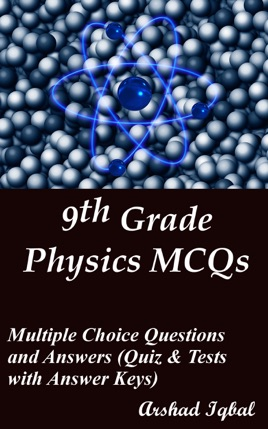• \$3.99

## Publisher Description

9th grade physics multiple choice questions has 239 MCQs. Grade 9 physics quiz questions and answers pdf, MCQs on dynamics, gravitation, kinematics, work, energy and power, forms of energy, specific heat capacity, thermal properties, heat, matter properties, what is physics MCQs with answers, earth mass, center of gravity, turning effect of forces, kinetic molecular theory, physical quantities and measurement MCQs and quiz worksheets to practice exam prep tests.
9th grade physics multiple choice quiz questions and answers pdf, physics exam revision and study guide with practice tests for online exam prep and interviews. Physics interview questions and answers to ask, to prepare and to study for jobs interviews and career MCQs with answer keys.
Dynamics quiz has 27 multiple choice questions. Gravitation quiz has 15 multiple choice questions. Kinematics quiz has 32 multiple choice questions with answers. Matter properties quiz has 32 multiple choice questions. Physical quantities and measurement quiz has 35 multiple choice questions. Thermal properties of matter quiz has 28 multiple choice questions. Transfer of heat quiz has 17 multiple choice questions. Turning effect of forces quiz has 28 multiple choice questions. Work and energy quiz has 25 multiple choice questions.
Physics interview questions and answers pdf, MCQs on power, couple, efficiency, thermometer, equilibrium, prefixes, kinetic energy, convection, scientific notation, conduction, evaporation, potential energy, significant figures, atmospheric pressure, Archimedes principle, elasticity, thermal expansion, newton’s laws of motion, gravitational force, mass of earth, specific heat capacity, center of gravity, forms of energy, kinetic molecular theory, sources of energy, equations of motion, center of mass, artificial satellites, types of motion, what is pressure, uniform circular motion, change of state, hooks law, physical quantities, scalars and vectors, international system of units, work and energy, introduction to physics, least count, principle of moments, resolution of forces, resolution of vectors, thermal properties of matter, parallel forces, temperature and heat, principle of floatation, rest and motion, addition of forces, heat and heat transfer, motion of free falling bodies, physics measuring instruments, matter density, analysis of motion, heat transfer and radiation, liquids pressure, dynamics and friction, force inertia and momentum, g value and altitude, motion key terms, radiations and applications, torque and moment of force, grade 9 physics worksheets for competitive exams preparation.